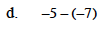### Home > MC1 > Chapter 3 > Lesson 3.4.3 > Problem3-118

3-118.
1. . Find the value of each expression. Change any subtraction problem to an equivalent addition problem and draw it with + and − pieces. Homework Help ✎

1. 5 − 7

2. −5 + (−7)

3. −5 + 7

4. −5 − (−7)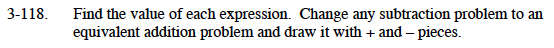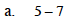The equivalent addition problem is 5 + (−7).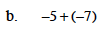A drawing should look something like this:

− − − − −
− − − − − − −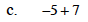2